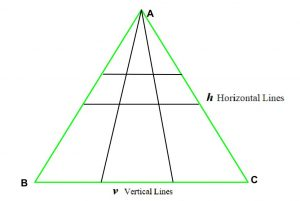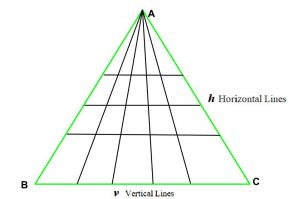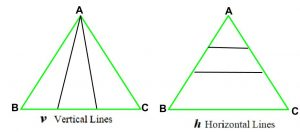Open in App
Not now

# Total number of triangles formed when there are H horizontal and V vertical lines

• Last Updated : 07 Apr, 2021

Given a triangle ABC. H horizontal lines from side AB to AC (as shown in fig.) and V vertical lines from vertex A to side BC are drawn, the task is to find the total no. of triangles formed.
Examples:

Input: H = 2, V = 2
Output: 18As we see in the image above, total triangles formed are 18.
Input: H = 3, V = 4
Output: 60Approach: As we see in the images below, we can derive a general formula for above problem:

1. If there are only h horizontal lines then total triangles are (h + 1).
2. If there are only v vertical lines then total triangles are (v + 1) * (v + 2) / 2.1. So, total triangles are Triangles formed by horizontal lines * Triangles formed by vertical lines i.e. (h + 1) * (( v + 1) * (v + 2) / 2).

Below is the implementation of the above approach:

## C++

 `// C++ implementation of the approach``#include ``using` `namespace` `std;``#define LLI long long int` `// Function to return total triangles``LLI totalTriangles(LLI h, LLI v)``{``    ``// Only possible triangle is``    ``// the given triangle``    ``if` `(h == 0 && v == 0)``        ``return` `1;` `    ``// If only vertical lines are present``    ``if` `(h == 0)``        ``return` `((v + 1) * (v + 2) / 2);` `    ``// If only horizontal lines are present``    ``if` `(v == 0)``        ``return` `(h + 1);` `    ``// Return total triangles``    ``LLI Total = (h + 1) * ((v + 1) * (v + 2) / 2);` `    ``return` `Total;``}` `// Driver code``int` `main()``{``    ``int` `h = 2, v = 2;``    ``cout << totalTriangles(h, v);` `    ``return` `0;``}`

## Java

 `// Java implementation of the approach``class` `GFG {` `    ``// Function to return total triangles``    ``public` `static` `int` `totalTriangles(``int` `h, ``int` `v)``    ``{``        ``// Only possible triangle is``        ``// the given triangle``        ``if` `(h == ``0` `&& v == ``0``)``            ``return` `1``;` `        ``// If only vertical lines are present``        ``if` `(h == ``0``)``            ``return` `((v + ``1``) * (v + ``2``) / ``2``);` `        ``// If only horizontal lines are present``        ``if` `(v == ``0``)``            ``return` `(h + ``1``);` `        ``// Return total triangles``        ``int` `total = (h + ``1``) * ((v + ``1``) * (v + ``2``) / ``2``);` `        ``return` `total;``    ``}` `    ``// Driver code``    ``public` `static` `void` `main(String[] args)``    ``{``        ``int` `h = ``2``, v = ``2``;``        ``System.out.print(totalTriangles(h, v));``    ``}``}`

## C#

 `// C# implementation of the approach``using` `System;` `class` `GFG``{` `    ``// Function to return total triangles``    ``public` `static` `int` `totalTriangles(``int` `h, ``int` `v)``    ``{``        ``// Only possible triangle is``        ``// the given triangle``        ``if` `(h == 0 && v == 0)``            ``return` `1;` `        ``// If only vertical lines are present``        ``if` `(h == 0)``            ``return` `((v + 1) * (v + 2) / 2);` `        ``// If only horizontal lines are present``        ``if` `(v == 0)``            ``return` `(h + 1);` `        ``// Return total triangles``        ``int` `total = (h + 1) * ((v + 1) * (v + 2) / 2);` `        ``return` `total;``    ``}` `    ``// Driver code``    ``public` `static` `void` `Main()``    ``{``        ``int` `h = 2, v = 2;``        ``Console.Write(totalTriangles(h, v));``    ``}``}` `// This code is contributed by Ryuga`

## Python3

 `# Python3 implementation of the approach` `# Function to return total triangles``def` `totalTriangles(h, v):``    ` `    ``# Only possible triangle is``    ``# the given triangle``    ``if` `(h ``=``=` `0` `and` `v ``=``=` `0``):``        ``return` `1` `    ``# If only vertical lines are present``    ``if` `(h ``=``=` `0``):``        ``return` `((v ``+` `1``) ``*` `(v ``+` `2``) ``/` `2``)` `    ``# If only horizontal lines are present``    ``if` `(v ``=``=` `0``):``        ``return` `(h ``+` `1``)` `    ``# Return total triangles``    ``total ``=` `(h ``+` `1``) ``*` `((v ``+` `1``) ``*` `(v ``+` `2``) ``/` `2``)` `    ``return` `total` `# Driver code``h ``=` `2``v ``=` `2``print``(``int``(totalTriangles(h, v)))`

## PHP

 ``

## Javascript

 ``

Output:

`18`

Time Complexity: O(1)
Auxiliary Space: O(1)

My Personal Notes arrow_drop_up